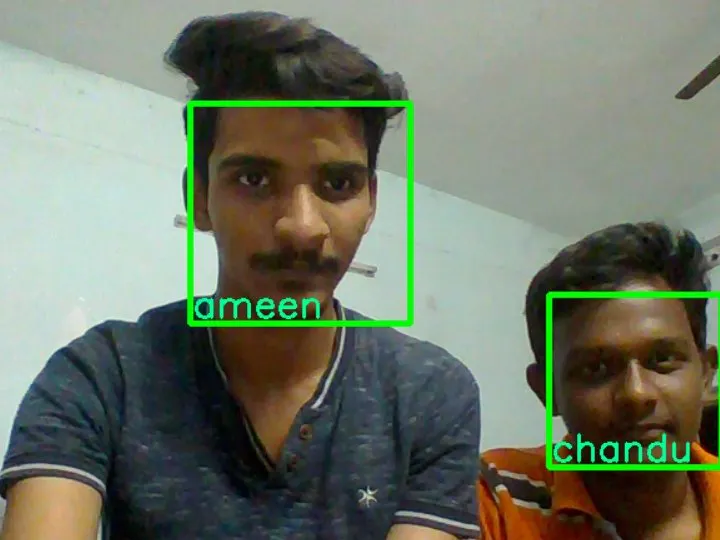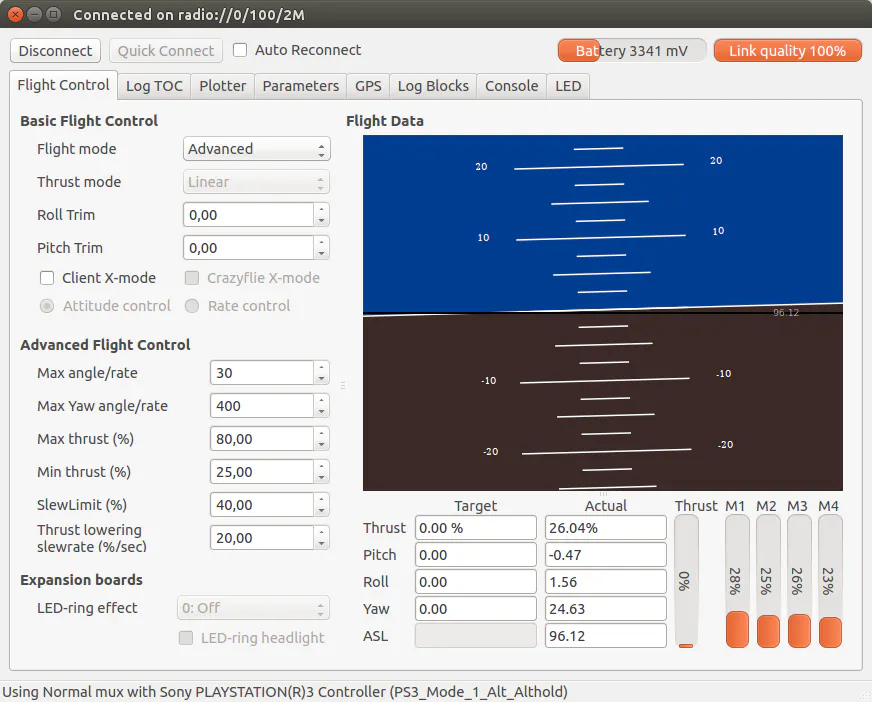# Face Detection and Recognition

Every robot needs to identify its owner.## Things used in this project

### Hardware componentsArduino UNO & Genuino UNO
×1

### Software apps and online servicesBitcraze Crazyflie Python Client

## Code

### Code snippet #9

Plain text
```import cv2

cap = cv2.VideoCapture("input_video.mp4")

print cap.isOpened()   # True = read video successfully. False - fail to read video.

fourcc = cv2.VideoWriter_fourcc(*'XVID')

out = cv2.VideoWriter("output_video.avi", fourcc, 20.0, (640, 360))

print out.isOpened()  # True = write out video successfully. False - fail to write out video.

cap.release()

out.release()
```

### Code snippet #10

Plain text
```import numpy as np

import cv2

cap = cv2.VideoCapture(0)<br>while 1:

gray = cv2.cvtColor(img, cv2.COLOR_BGR2GRAY)

for (x,y,w,h) in faces:

cv2.rectangle(img,(x,y),(x+w,y+h),(255,0,0),2)

roi_gray = gray[y:y+h, x:x+w]

roi_color = img[y:y+h, x:x+w]

for (ex,ey,ew,eh) in eyes:

cv2.rectangle(roi_color,(ex,ey),(ex+ew,ey+eh),(0,255,0),2)

print "found " +str(len(faces)) +" face(s)"

cv2.imshow('img',img)

k = cv2.waitKey(30) & 0xff

if k == 27:

break

cap.release()

cv2.destroyAllWindows()
```

### Code snippet #11

Plain text
```import numpy as np

import cv2

cap = cv2.VideoCapture(0)

id = raw_input('enter user id')

sampleN=0;

while 1:

gray = cv2.cvtColor(img, cv2.COLOR_BGR2GRAY)

for (x,y,w,h) in faces:

sampleN=sampleN+1;

cv2.imwrite("F:/Program Files/projects/face_rec/facesData/User."+str(id)+ "." +str(sampleN)+ ".jpg", gray[y:y+h, x:x+w])

cv2.rectangle(img,(x,y),(x+w,y+h),(255,0,0),2)

cv2.waitKey(100)

cv2.imshow('img',img)

cv2.waitKey(1)

if sampleN > 20:

break

cap.release()

cv2.destroyAllWindows()
```

### Code snippet #13

Plain text
```import os

import numpy as np

import cv2

from PIL import Image # For face recognition we will the the LBPH Face Recognizer

recognizer = cv2.createLBPHFaceRecognizer();

path="F:/Program Files/projects/face_rec/facesData"

def getImagesWithID(path):

imagePaths = [os.path.join(path, f) for f in os.listdir(path)]

# print image_path

#getImagesWithID(path)

faces = []

IDs = []

for imagePath in imagePaths:

# Read the image and convert to grayscale

facesImg = Image.open(imagePath).convert('L')

faceNP = np.array(facesImg, 'uint8')

# Get the label of the image

ID= int(os.path.split(imagePath)[-1].split("."))

# Detect the face in the image

faces.append(faceNP)

IDs.append(ID)

cv2.waitKey(10)

return np.array(IDs), faces

Ids,faces  = getImagesWithID(path)

recognizer.train(faces,Ids)

recognizer.save("F:/Program Files/projects/face_rec/faceREC/trainingdata.yml")

cv2.destroyAllWindows()
```

### Code snippet #14

Plain text
```import numpy as npimport cv2
cap = cv2.VideoCapture(0)
rec = cv2.createLBPHFaceRecognizer();
id=0
font=cv2.cv.InitFont(cv2.cv.CV_FONT_HERSHEY_COMPLEX_SMALL,5,1,0,4)
while 1:
gray = cv2.cvtColor(img, cv2.COLOR_BGR2GRAY)
for (x,y,w,h) in faces:
cv2.rectangle(img,(x,y),(x+w,y+h),(255,0,0),2)
id,conf=rec.predict(gray[y:y+h,x:x+w])
if(id==2):
id="alok"
if id==1:
id="alok"
if id==3:
id="anjali"
if id==4:
id="Gaurav"
if id==5:
id='rahul'
if id==6:
id="akshay"
cv2.cv.PutText(cv2.cv.fromarray(img),str(id),(x,y+h),font,255)
cv2.imshow('img',img)

if cv2.waitKey(1) == ord('q'):
break
cap.release()cv2.destroyAllWindows()
```